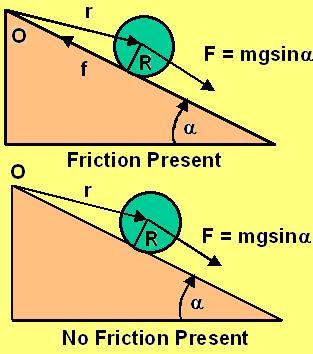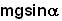Monday, June 30, 2008

Irodov Problem 1.195The sphere is acted upon by two forces i) the force of gravity mg whose component along the inclined plane isand ii) the force of friction acting at the point of contact between the inclined plane and the sphere. As seen from the initial point of contact O there is however only one torque acting on the system - that due to the force of gravity given by. The force of friction f acting on the sphere does not induce a torque on the sphere as seen from O since it passes through the point O so that moment arm for the friction force is 0.

Torque is the rate of change of angular momentum and so if the angular momentum of the sphere at any point is M, then,Clearly the absence or presence of friction does not alter anything since it has no net torque on the sphere as seen from point O.

Unknown said...

The explanation provided (that the frictional force does not produce any torque about point O) appears alright, but look at the problem from a different perspective.

For the rolling sphere, first compute the linear momentum(P) at time 't' which is equal to (5/7)mgtsin(alpha) and then take its moment about point O. The moment arm is R and therefore the angular momentum about O is (5/7)Rmgt sin(alpha).

This is different from your answer. Why the discrepancy and where is the error? I'm keen to know.

Unknown said...

I had posted a comment/doubt this afternoon but later I got the issue sorted out.

The answer lies in Problem 1.197 itself - I should have added the angular momentum of the rolling sphere about its centre of mass -(2/7)Rmgt sin(alpha)giving a total angular momentum about point O which is same as Rmgt sin(alpha).